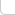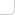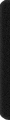# 某岛

… : "…アッカリ～ン . .. . " .. .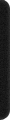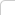April 12, 2013

# Codeforces Round #179

## Problem A. Greg and Array

。。略）

### Analysis:

。。其实这题不需要使用线段树或是树状数组。。。
。。。对这类各种操作。。一次询问的题总有更直接的处理方法。。

。。参见咖啡枢纽。。。

## Problem C. Greg and Friends

### Brief description:

。。n 个质量分别为 1, 2 的人驾驶着一艘载重为 k 的小船渡河。
。。问把人全部送到对岸的最少往返次数。。。以及满足最少往返次数条件下的方案数。。
( n = 50）。。

### Analysis:

… 个人认为本题十分 Nice。。。很容易想到 dp[往返次数] 然后枚举  的人数这种五次方 DP。。。
。。。。比赛时总会有人误认为。。返回的时候一定只搭乘1个人才能最优。。。。
。。实际上继续观察我们会发现。。往返这两个过程。。并没有本质上的差异。。。应写成统一形式。。。。

```const int N = 59;
Int f[N][N], C[N][N];
int n, k, p, q, c1, c2;

void Prep(){
swap(p, q); REP_2(a1, a2, c1+1, c2+1) f[p][a1][a2] = 0;
}

#define b (b1+b2*2)

void Roll(){
Prep(); REP_2(a1, a2, c1+1, c2+1) if (f[q][a1][a2]) REP_2(b1, b2, a1+1, a2+1) if (b &&b <= k){
f[p][c1-a1+b1][c2-a2+b2] += C[a1][b1] * C[a2][b2] * f[q][a1][a2];
}
}

int main(){

#ifndef ONLINE_JUDGE
freopen("in.txt", "r", stdin);
//freopen("out.txt", "w", stdout);
#endif

RD(n, k); k /= 50; DO(n) if (RD()==50) ++c1; else ++c2;
REP(i, n+1){C[i] = 1; REP_1(j, i) C[i][j] = C[i-1][j-1] + C[i-1][j];}

p = 0, q = 1; Prep(); f[p][c1][c2] = 1; REP(t, 2*n){

Roll();

if (f[p][c1][c2]){
printf("%d\n%d", t*2+1, int(f[p][c1][c2]));
return 0;
}

Roll();
}

printf("-1\n0");
}
```

## Problem D. Greg and Caves

### Brief description:

。。图案是凸的。。上下的宽度至少是 2.。。。
。。且中间存在一个梗。（可以覆盖其他所有行）。。
( n,m = 2000)

### Analysis:

。。大致也可以归类到培养皿问题。。但是因为中间有一个梗而且没有障碍。。

。。所以可以 dp 出一个方向的然后连起来。。。
。。f[][] 表示高度恰好为[]。。梗的宽度为 []。。

```1 1 1 1 1 1 1
1 3 6 10 15 21 28
1 5 15 35 70 126 210
1 7 28 84 210 462 924
```

```C(0, 0) C(1, 1) C(2, 2) C(3, 3)
C(2, 0) C(3, 1) C(4, 2) C(5, 3)
C(4, 0) C(5, 1) C(6, 2) C(7, 3)
C(6, 0) C(7, 1) C(8, 2) C(9, 3)
```

。。。应该可以联系挡板什么的给出个比较合理的组合解释。。。反正我是怎么都不行。。
。。然后 s[][] 表示高度至多为 []。。然后宽度为 []。。。。
。。连起来的时候用 f[][] 和 s[][] ×起来就好。

。s[][] 可以看成是浮动窗口。。从而不会引起重复计数。。。

```const int N = 2009;
Int f[N][N], s[N][N];
int n, m;

int main(){

#ifndef ONLINE_JUDGE
freopen("in.txt", "r", stdin);
//freopen("out.txt", "w", stdout);
#endif

RD(n, m);

FOR_1(i, 2, m) f[i] = 1; FOR_1(i, 2, n){Int s = 0; FOR_1(j, 2, m) f[i][j] = (s += f[i-1][j]) + f[i][j-1];}
CPY(s, f); FOR_1(i, 2, n) FOR_1(j, 2, m) s[i][j] += s[i-1][j];
Int res = 0; REP_1(i, 1) FOR_1(j, 2, m) res += s[i][j] * f[n-i+1][j] * (m-j+1);

OT(res);
}
```

## Problem E. Yaroslav and Points

### Brief description:

。。x 轴 上分布着 n 个点。。要求支持以下两个操作。。

• 询问所有范围 [l, r] 之间的点对的距离的和。
• 移动其中一个点的坐标。

### Analysis:

… 似乎方法很多。。不过果然还是 Splay 最好弄了。。。。

```/*
x
[l] [r]
*/
inline void update(){
sz = l->sz + 1 + r->sz, s = l->s + ky + r->s; // size, sum ..
ss = l->ss + (l->sz*ky-l->s) + (l->sz*r->s - r->sz*l->s) + (r->s-r->sz*ky) + r->ss; // 点对和
//      x 点和左子树。。。     //  跨越 x 点的。。        // 和右子树。。
}
```

。。对于询问操作而言。。。是可以直接在 splay 里面进行查找的。。但是要写成 lower_bound upper_bound 那种着实比较麻烦。。
。。而修改操作。。也需要查找某个点的位置。。而且考虑到没有重复结点。。写成前驱后继的话更不容易出错。。

（。。xwlj 的 E 题大裸数据结构要不要这样。。codeforces 果然也开始世风日下了么。。
http://codeforces.com/contest/295/submission/3515011